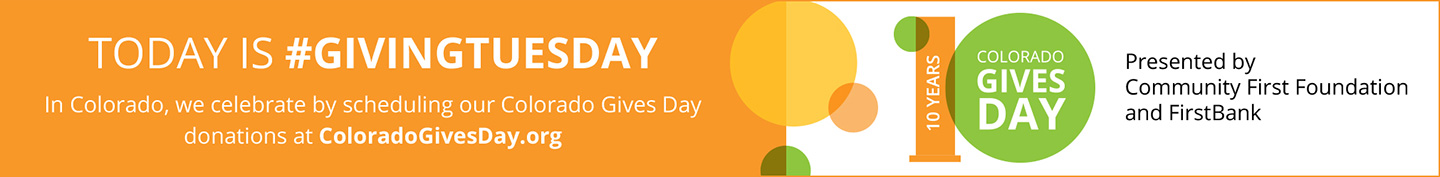# BITSAT 2022 Physics Syllabus

1. Units & Measurement

• Units (Different systems of units, SI units, fundamental and derived units)
• Dimensional Analysis
• Precision and significant figures
• Fundamental measurements in Physics (Vernier calipers, screw gauge, Physical balance etc)

2. Kinematics

• Properties of vectors
• Position, velocity and acceleration vectors
• Motion with constant acceleration
• Projectile motion
• Uniform circular motion
• Relative motion

3. Newton’s Laws of Motion

• Newton’s laws (free body diagram, resolution of forces)
• Motion on an inclined plane
• Motion of blocks with pulley systems
• Circular motion – centripetal force
• Inertial and non-inertial frames

4. Impulse and Momentum

• Definition of impulse and momentum
• Conservation of momentum
• Collisions
• Momentum of a system of particles
• Center of mass

5. Work and Energy

• Work done by a force
• Kinetic energy and work-energy theorem
• Power
• Conservative forces and potential energy
• Conservation of mechanical energy

6. Rotational Motion

• Description of rotation (angular displacement, angular velocity and angular acceleration)
• Rotational motion with constant angular acceleration
• Moment of inertia, Parallel and perpendicular axes theorems, rotational kinetic energy6.4 Torque and angular momentum
• Conservation of angular momentum
• Rolling motion

7. Gravitation

• Newton’s law of gravitation
• Gravitational potential energy, Escape velocity
• Motion of planets – Kepler’s laws, satellite motion

8. Mechanics of Solids  and Fluids

• Elasticity
• Pressure, density and Archimedes’ principle
• Viscosity and Surface Tension
• Bernoulli’s theorem

9. Oscillations

• Kinematics of simple harmonic motion
• Spring mass system, simple and compound pendulum
• Forced & damped oscillations, resonance

10. Waves

• Progressive sinusoidal waves
• Standing waves in strings and pipes
• Superposition of waves, beats
• Doppler Effect

11. Heat and Thermodynamics

• Kinetic theory of gases
• Thermal equilibrium and temperature
• Specific heat, Heat Transfer – Conduction, convection and radiation, thermal conductivity, Newton’s law of cooling
• Work, heat and first law of thermodynamics
• 2nd law of thermodynamics, Carnot engine – Efficiency and Coefficient of performance

12. Electrostatics

• Coulomb’s law
• Electric field (discrete and continuous charge distributions)
• Electrostatic potential and Electrostatic potential energy
• Gauss’ law and its applications
• Electric dipole
• Capacitance and dielectrics (parallel plate capacitor, capacitors in series and parallel)

13.Current Electricity

• Ohm’s law, Joule heating
• D.C circuits – Resistors and cells in series and parallel, Kirchoff’s laws, potentiometer and Wheatstone bridge,
• Electrical Resistance (Resistivity, origin and temperature dependence of resistivity).

14. Magnetic Effect of Current

• Biot-Savart’s law and its applications
• Ampere’s law and its applications
• Lorentz force, force on current carrying conductors in a magnetic field
• Magnetic moment of a current loop, torque on a current loop, Galvanometer and its conversion to voltmeter and ammeter

15. Electromagnetic Induction

• Faraday’s law, Lenz’s law, eddy currents
• Self and mutual inductance
• Transformers and generators
• Alternating current (peak and rms value)
• AC circuits, LCR circuits

16. Optics

• Laws of reflection and refraction
• Lenses and mirrors
• Optical instruments – telescope and microscope
• Interference – Huygen’s principle, Young’s double slit experiment
• Interference in thin films
• Diffraction due to a single slit
• Electromagnetic waves and their characteristics (only qualitative ideas), Electromagnetic spectrum
• Polarization – states of polarization, Malus’ law, Brewster’s law

17. Modern Physics

• Dual nature of light and matter – Photoelectric effect. De Broglie wavelength
• Atomic models – Rutherford’s experiment, Bohr’s atomic model
• Hydrogen atom spectrum
• Nuclear reactions : Fission and fusion, binding energy

18. Electronic Devices

• Energy bands in solids (qualitative ideas only), conductors, insulators and semiconductors;
• Semiconductor d iode- I-V characteristics of a transistor; transistor as an amplifier (common emitter configuration) and oscillator.
• Logic rates (OR, AND, NOT, NAND and NOR). Transistor as a switch.